# specifyCoefficients

Specify coefficients in PDE model

## Syntax

``specifyCoefficients(model,m=m,d=d,c=c,a=a,f=f)``
``specifyCoefficients(model,m=m,d=d,c=c,a=a,f=f,RegionType=RegionID)``
``CA = specifyCoefficients(___)``

## Description

example

````specifyCoefficients(model,m=m,d=d,c=c,a=a,f=f)` defines the PDE coefficients and includes them in `model`. You must specify coefficients: `m`, `d`, `c`, `a`, and `f`. This syntax applies coefficients to the entire geometry. NoteInclude a geometry in `model` before using `specifyCoefficients`. ```

example

````specifyCoefficients(model,m=m,d=d,c=c,a=a,f=f,RegionType=RegionID)` assigns coefficients for a specified geometry region.```

example

````CA = specifyCoefficients(___)` returns a handle to the coefficient assignment object in `model`.```

## Examples

collapse all

Specify the coefficients for Poisson's equation $-\nabla \cdot \nabla u=1$.

`solvepde` addresses equations of the form

$m\frac{{\partial }^{2}u}{\partial {t}^{2}}+d\frac{\partial u}{\partial t}-\nabla \cdot \left(c\nabla u\right)+au=f$.

Therefore, the coefficients for Poisson's equation are $m=0$, $d=0$, $c=1$, $a=0$, $f=1$. Include these coefficients in a PDE model of the L-shaped membrane.

```model = createpde(); geometryFromEdges(model,@lshapeg); specifyCoefficients(model,"m",0,... "d",0,... "c",1,... "a",0,... "f",1);```

Specify zero Dirichlet boundary conditions, mesh the model, and solve the PDE.

```applyBoundaryCondition(model,"dirichlet", ... "Edge",1:model.Geometry.NumEdges, ... "u",0); generateMesh(model,"Hmax",0.25); results = solvepde(model);```

View the solution.

`pdeplot(model,"XYData",results.NodalSolution)`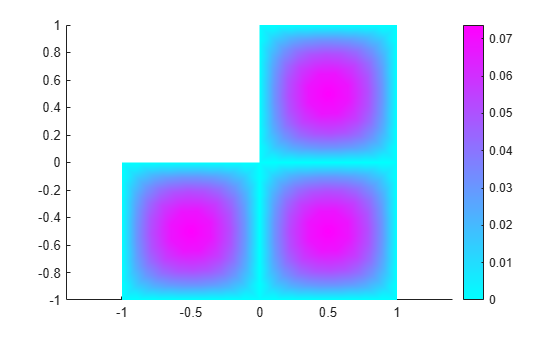Specify coefficients for Poisson's equation in 3-D with a nonconstant source term, and obtain the coefficient object.

The equation coefficients are $m=0$, $d=0$, $c=1$, $a=0$. For the nonconstant source term, take $f={y}^{2}\mathrm{tanh}\left(z\right)/1000$.

`f = @(location,state)location.y.^2.*tanh(location.z)/1000;`

Set the coefficients in a 3-D rectangular block geometry.

```model = createpde(); importGeometry(model,"Block.stl"); CA = specifyCoefficients(model,"m",0,... "d",0,... "c",1,... "a",0,... "f",f)```
```CA = CoefficientAssignment with properties: RegionType: 'cell' RegionID: 1 m: 0 d: 0 c: 1 a: 0 f: @(location,state)location.y.^2.*tanh(location.z)/1000 ```

Set zero Dirichlet conditions on face 1, mesh the geometry, and solve the PDE.

```applyBoundaryCondition(model,"dirichlet","Face",1,"u",0); generateMesh(model); results = solvepde(model);```

View the solution on the surface.

`pdeplot3D(model,"ColorMapData",results.NodalSolution)`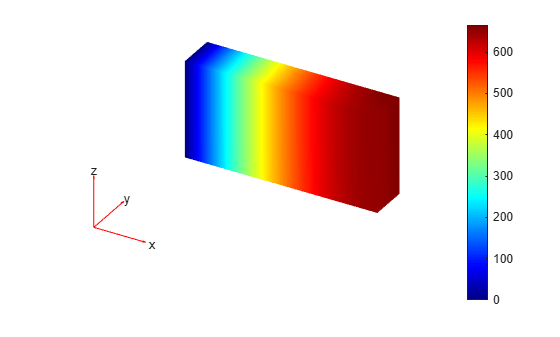Create a scalar PDE model with the L-shaped membrane as the geometry. Plot the geometry and subdomain labels.

```model = createpde(); geometryFromEdges(model,@lshapeg); pdegplot(model,"FaceLabels","on") axis equal ylim([-1.1,1.1])```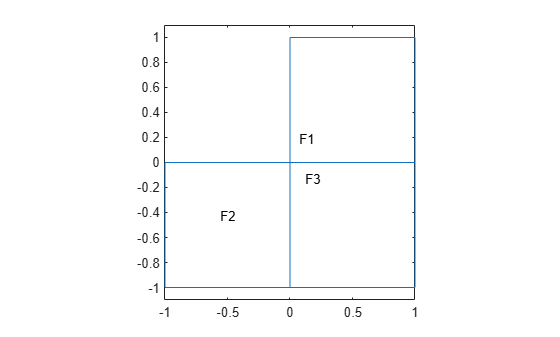Set the `c` coefficient to 1 in all domains, but the `f` coefficient to 1 in subdomain 1, 5 in subdomain 2, and -8 in subdomain 3. Set all other coefficients to 0.

```specifyCoefficients(model,"m",0,"d",0,"c",1,"a",0,"f",1,"Face",1); specifyCoefficients(model,"m",0,"d",0,"c",1,"a",0,"f",5,"Face",2); specifyCoefficients(model,"m",0,"d",0,"c",1,"a",0,"f",-8,"Face",3);```

Set zero Dirichlet boundary conditions to all edges. Create a mesh, solve the PDE, and plot the result.

```applyBoundaryCondition(model,"dirichlet", ... "Edge",1:model.Geometry.NumEdges, ... "u",0); generateMesh(model,"Hmax",0.25); results = solvepde(model); pdeplot(model,"XYData",results.NodalSolution)```Perform transient analysis of a simple cantilever beam with and without damping. To include damping in the analysis, specify nonzero `m` and `d` coefficients.

Create a geometry representing a cantilever beam. The beam is 5 inches long and 0.1 inch thick.

```height = 0.1; width = 5; gm = [3;4;0;width;width;0;0;0;height;height]; g = decsg(gm,'S1',('S1')');```

Create a `PDEModel` object with two independent variables, and include a geometry in the model.

```model = createpde(2); geometryFromEdges(model,g);```

Plot the geometry with edge labels.

`pdegplot(model,EdgeLabels="on")`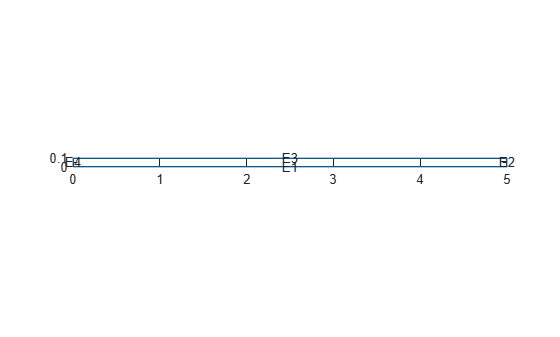Specify that the left edge of the beam is a fixed boundary.

`applyBoundaryCondition(model,"dirichlet",Edge=4,u=[0,0]);`

Specify Young's modulus, Poisson's ratio, and the mass density of the beam assuming that it is made of steel.

```E = 3.0e7; nu = 0.3; rho = 0.3/386;```

Specify the PDE coefficients for the undamped model by setting the `d` coefficient to 0.

```G = E/(2.*(1+nu)); mu = 2.0*G*nu/(1-nu); specifyCoefficients(model, ... m=rho, ... d=0, ... c=[2*G+mu;0;G;0;G;mu;0;G;0;2*G+mu], ... a=0, ... f=[0;0]);```

Set the initial displacement to $\left(0;{10}^{-4}{\mathit{x}}^{2}\right)$ and the initial velocity to (0; 0).

`setInitialConditions(model,@(location) location.x.^2.*[0;0.0001],[0;0]);`

Generate a mesh and solve the problem.

```generateMesh(model); tlist = 0:0.25/1000:0.25; tres = solvepde(model,tlist);```

Plot the undamped solution.

```uu = tres.NodalSolution; utip = uu(2,2,:); plot(tlist,utip(:))```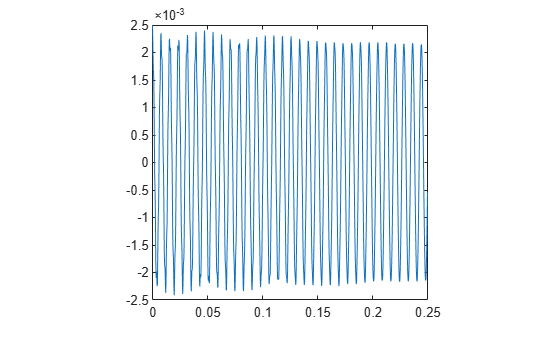Obtain assembled mass and stiffness matrices by calling `assembleFEMatrices`.

`fem = assembleFEMatrices(model);`

Now specify the coefficients for the beam model with Rayleigh damping. The `d` coefficient represents the damping matrix. The `d` coefficient is a linear combination of the mass matrix `fem.M` and the stiffness matrix `fem.K`.

```alpha = 10; beta = 0; dampmat = alpha*fem.M + beta*fem.K; specifyCoefficients(model, ... m=rho, ... d=dampmat, ... c=[2*G+mu;0;G;0;G;mu;0;G;0;2*G+mu], ... a=0, ... f=[0;0]);```

Solve the problem and plot the damped solution.

```tres = solvepde(model,tlist); uu = tres.NodalSolution; utip = uu(2,2,:); plot(tlist,utip(:))```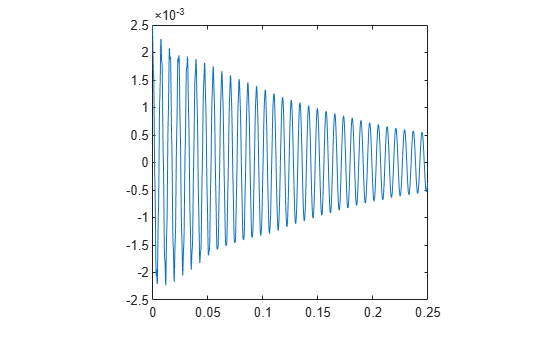## Input Arguments

collapse all

PDE model, specified as a `PDEModel` object.

Example: `model = createpde`

Second-order time derivative coefficient, specified as a scalar, column vector, or function handle. For details on the sizes, and for details of the function handle form of the coefficient, see m, d, or a Coefficient for specifyCoefficients.

Specify 0 on the entire geometry if the term is not part of your problem. If this coefficient is zero in one subdomain of the geometry, it must be zero on the entire geometry.

Example: `specifyCoefficients("m",@mcoef,"d",0,"c",1,"a",0,"f",1,"Face",1:4)`

Data Types: `double` | `function_handle`
Complex Number Support: Yes

First-order time derivative coefficient, specified as a scalar, column vector, or function handle. For details on the sizes, and for details of the function handle form of the coefficient, see m, d, or a Coefficient for specifyCoefficients.

Note

If the `m` coefficient is nonzero, `d` must be `0` or a matrix, and not a function handle. See d Coefficient When m Is Nonzero.

Specify 0 on the entire geometry if the term is not part of your problem. If this coefficient is zero in one subdomain of the geometry, it must be zero on the entire geometry.

Example: `specifyCoefficients("m",0,"d",@dcoef,"c",1,"a",0,"f",1,"Face",1:4)`

Data Types: `double` | `function_handle`
Complex Number Support: Yes

Second-order space derivative coefficient, specified as a scalar, column vector, or function handle. For details on the sizes, and for details of the function handle form of the coefficient, see c Coefficient for specifyCoefficients.

This coefficient must not be zero in one subdomain of the geometry while nonzero in another subdomain.

Example: `specifyCoefficients("m",0,"d",0,"c",@ccoef,"a",0,"f",1,"Face",1:4)`

Data Types: `double` | `function_handle`
Complex Number Support: Yes

Solution multiplier coefficient, specified as a scalar, column vector, or function handle. For details on the sizes, and for details of the function handle form of the coefficient, see m, d, or a Coefficient for specifyCoefficients.

Specify `0` if the term is not part of your problem. This coefficient can be zero in one subdomain and nonzero in another.

Example: `specifyCoefficients("m",0,"d",0,"c",1,"a",@acoef,"f",1,"Face",1:4)`

Data Types: `double` | `function_handle`
Complex Number Support: Yes

Source coefficient, specified as a scalar, column vector, or function handle. For details on the sizes, and for details of the function handle form of the coefficient, see f Coefficient for specifyCoefficients.

Specify `0` if the term is not part of your problem. This coefficient can be zero in one subdomain and nonzero in another.

Example: `specifyCoefficients("m",0,"d",0,"c",1,"a",0,"f",@fcoeff,"Face",1:4)`

Data Types: `double` | `function_handle`
Complex Number Support: Yes

Geometric region type, specified as `Face` or `Cell`.

Example: `specifyCoefficients(m=0,d=0,c=1,a=0,f=10,Cell=2)`

Data Types: `char` | `string`

Geometric region ID, specified as a positive integer or vector of positive integers. Find the region IDs by using `pdegplot`.

Example: `specifyCoefficients(m=0,d=0,c=1,a=0,f=10,Cell=1:3)`

Data Types: `double`

## Output Arguments

collapse all

Coefficient assignment, returned as a `CoefficientAssignment` object. See CoefficientAssignment Properties.

collapse all

### PDE Coefficients

`solvepde` solves PDEs of the form

`$m\frac{{\partial }^{2}u}{\partial {t}^{2}}+d\frac{\partial u}{\partial t}-\nabla ·\left(c\nabla u\right)+au=f$`

`solvepdeeig` solves PDE eigenvalue problems of the form

`$\begin{array}{l}-\nabla ·\left(c\nabla u\right)+au=\lambda du\\ \text{or}\\ -\nabla ·\left(c\nabla u\right)+au={\lambda }^{2}mu\end{array}$`

`specifyCoefficients` defines the coefficients m, d, c, a, and f in the PDE model.

### `d` Coefficient When `m` Is Nonzero

The `d` coefficient takes a special matrix form when `m` is nonzero. You must specify `d` as a matrix of a particular size, not as a function handle.

In the case of nonzero `m`, the `d` coefficient represents a damping matrix. To specify `d`, perform these two steps:

1. Assemble finite element matrices for the PDE problem with your original coefficients and `d` = 0 by calling `assembleFEMatrices`. Use the default `"none"` method for `assembleFEMatrices`.

```model = createpde(); geometryFromEdges(model,@lshapeg); generateMesh(model,Hmax=0.25); specifyCoefficients(model,m=1,d=0,c=1,a=0,f=0,Face=1); specifyCoefficients(model,m=1,d=0,c=1,a=0,f=2,Face=2); specifyCoefficients(model,m=1,d=0,c=1,a=0,f=-8,Face=3); results = assembleFEMatrices(model);```
2. Define the `d` coefficient as a matrix of size `results.M`. Generally, `d` is either proportional to `results.M` or is a linear combination of `results.M` and `results.K`.

```specifyCoefficients(model,m=1,d=0*results.M,c=1,a=0,f=0,Face=1); specifyCoefficients(model,m=1,d=1*results.M, ... % nonzero d c=1,a=0,f=2,Face=2); specifyCoefficients(model,m=1,d=0*results.M,c=1,a=0,f=-8,Face=3);```

For an example with nonzero `m` and `d` coefficients, see Damped Cantilever Beam.

## Tips

• For eigenvalue equations, the coefficients cannot depend on the solution `u` or its gradient.

• You can transform a partial differential equation into the required form by using Symbolic Math Toolbox™. The `pdeCoefficients` (Symbolic Math Toolbox) function converts a PDE into the required form and extracts the coefficients into a structure that can be used by `specifyCoefficients`.

The `pdeCoefficients` function also can return a structure of symbolic expressions, in which case you need to use `pdeCoefficientsToDouble` (Symbolic Math Toolbox) to convert these expressions to `double` format before passing them to `specifyCoefficients`.

## Version History

Introduced in R2016a